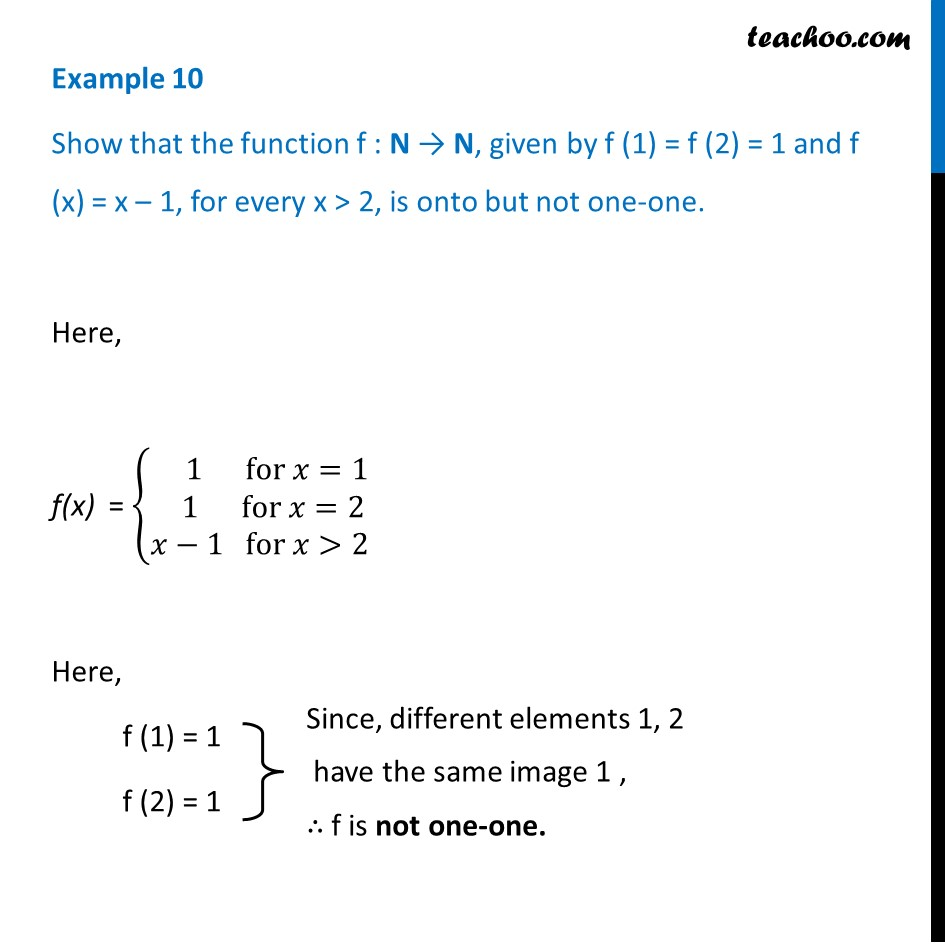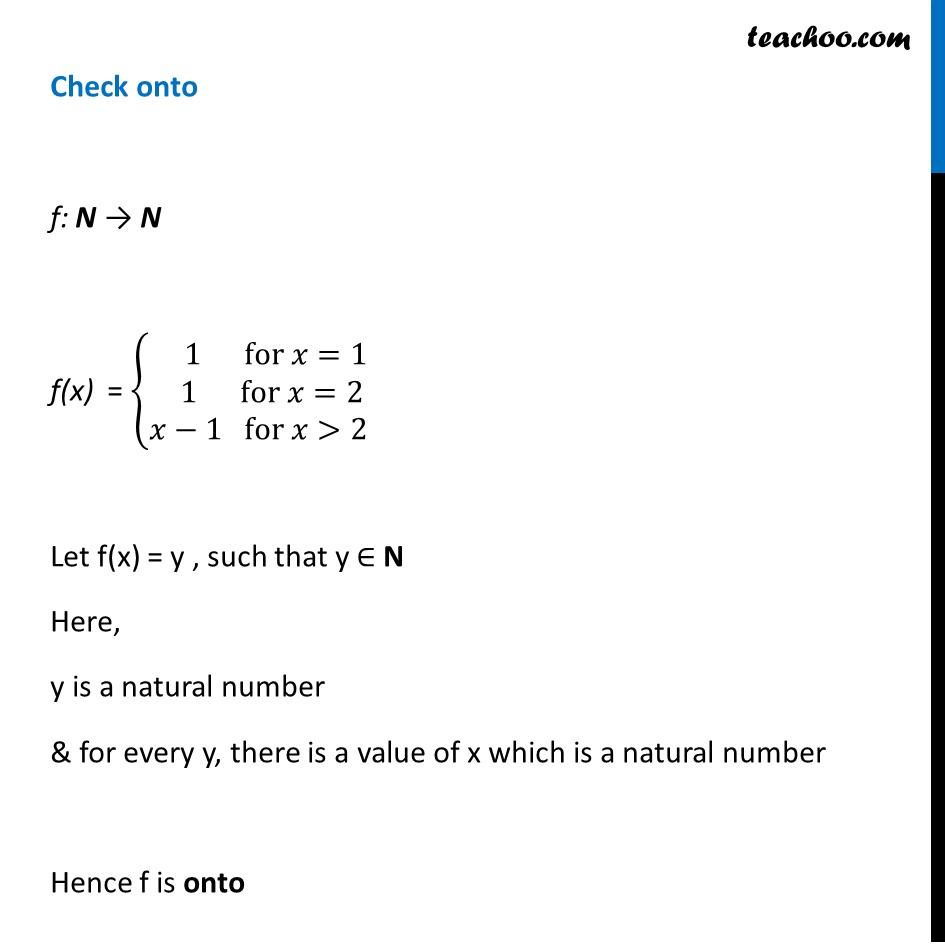Examples

Chapter 1 Class 12 Relation and Functions
Serial order wiseLearn in your speed, with individual attention - Teachoo Maths 1-on-1 Class

### Transcript

Example 10 Show that the function f : N → N, given by f (1) = f (2) = 1 and f (x) = x – 1, for every x > 2, is onto but not one-one. Here, f(x) = {█( 1 for 𝑥=1@ 1 for 𝑥=2@𝑥−1 for 𝑥>2)┤ Here, f (1) = 1 f (2) = 1 Check onto f: N → N f(x) = {█( 1 for 𝑥=1@ 1 for 𝑥=2@𝑥−1 for 𝑥>2)┤ Let f(x) = y , such that y ∈ N Here, y is a natural number & for every y, there is a value of x which is a natural number Hence f is onto